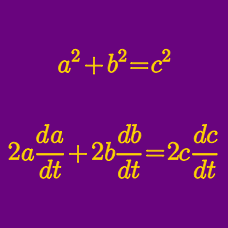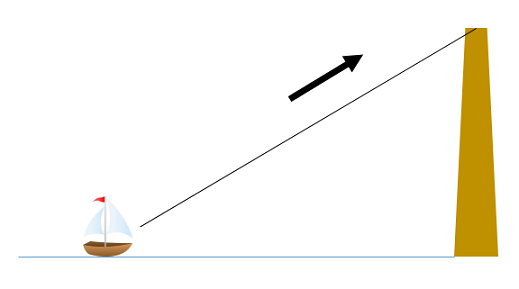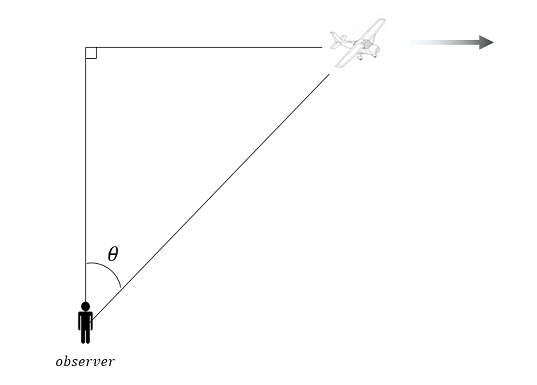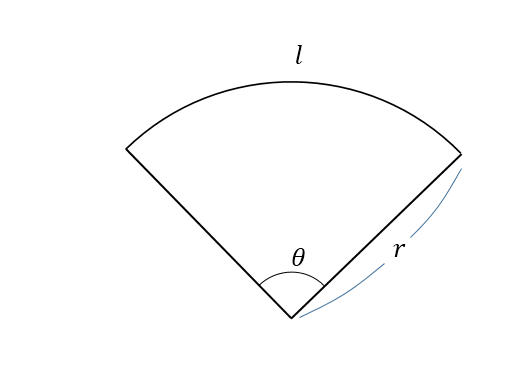Calculus

# Related Rates - 2D GeometryAs shown in the above diagram, a sailboat is pulled toward a rock face $30\text{m}$ high by a rope $66 \text{m}$ long. If the rope is pulled at a rate of $8 \text{m}$ per second, what is the speed of the sailboat after $2$ seconds?

Suppose a $20 \text{ cm} \times 20 \text{ cm}$ rectangle is modified such that the width of a rectangle increases at a rate of $10$ cm per second, while the length of the rectangle decreases at a rate of $3$ cm per second. What is the ratio $\text{Width} : \text{Length}$ when the rate of change of the area of the rectangle is $0?$A plane is flying in a straight line parallel to the ground at a constant speed of $400 \text{ m}$ per second at an altitude of $4000\text{ m},$ directly above an observer on the ground. After $3$ seconds, as shown in the above diagram, the observer now looks at the plane at an angle of $\theta$ from vertical. If the rate of change of $\theta$ with respect to time measured in seconds at this instant is $\displaystyle{\frac{d\theta}{dt}=\frac{a}{b}},$ where $a$ and $b$ are positive, coprime integers, what is $a+b?$

The radius of a circle starts at $10\text{ cm}$ and increases at the rate of $1\text{ mm}$ per second. If $S(t)$ is the area of the circle (in $\text{cm}^2$) after $t$ seconds and $S'(14) = a\pi \text{ cm}^2\text{/s},$ what is $a?$Consider a circular sector with radius $r$, central angle $\theta,$ and arc length $l$. If the circular sector begins to expand such that $\frac{dr}{dt} =8, \frac{d\theta}{dt}=7,$ what is $\displaystyle{ \frac{dl}{dt}}$ for $\displaystyle{ \theta = \frac{\pi}{2}}$ and $r = 15?$

×